# Boublik 2D hard convex body equation of state

Boublik 2D hard convex body equation of state 

Helmholtz energy function (Eq. 8):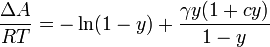$\frac{\Delta A}{RT} = - \ln (1-y) + \frac{\gamma y (1+cy)}{1-y}$

where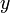$y$ is the packing fraction and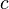$c$ is an unknown parameter and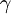$\gamma$ is the non-sphericity factor.

Compressibility factor (Eq. 9)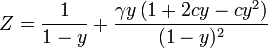$Z = \frac{ 1 }{1-y } + \frac{\gamma y \left( 1+2cy - cy^2\right) }{(1-y)^2}$

For the hard disk model (Eq. 11)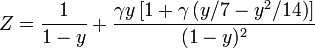$Z = \frac{ 1 }{1-y } + \frac{\gamma y \left[ 1+ \gamma \left(y/7 - y^2/14 \right) \right]}{(1-y)^2}$

where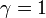$\gamma =1$